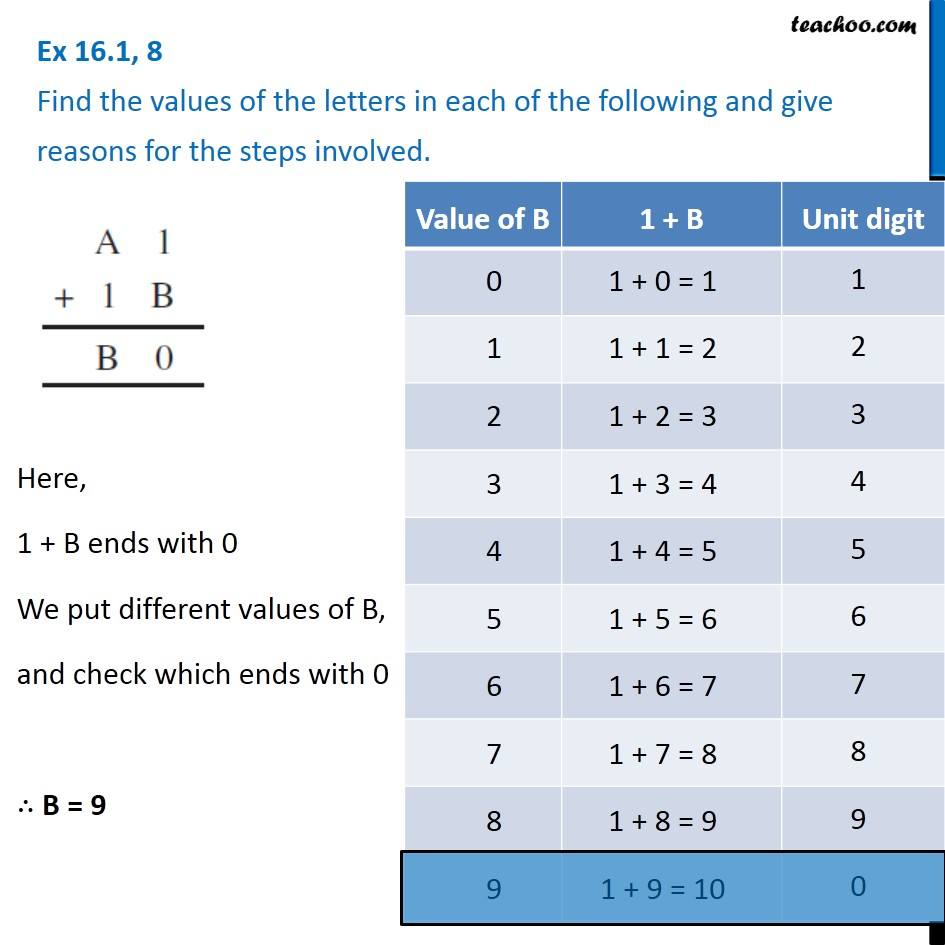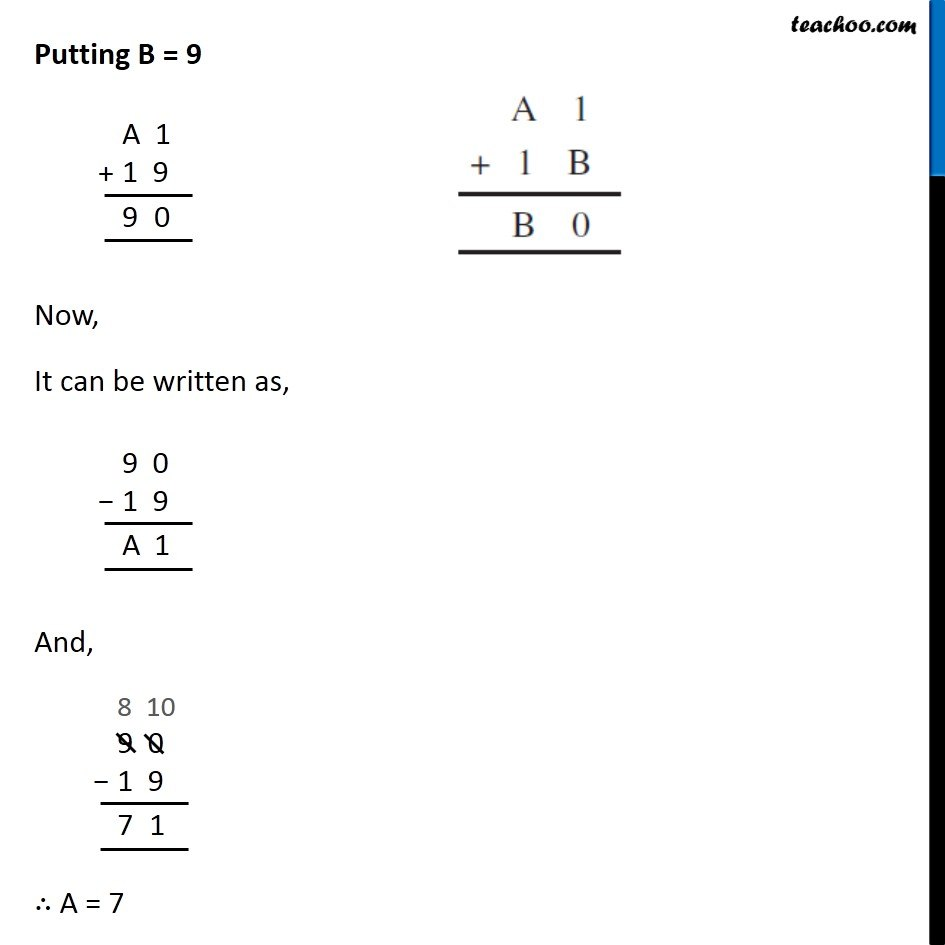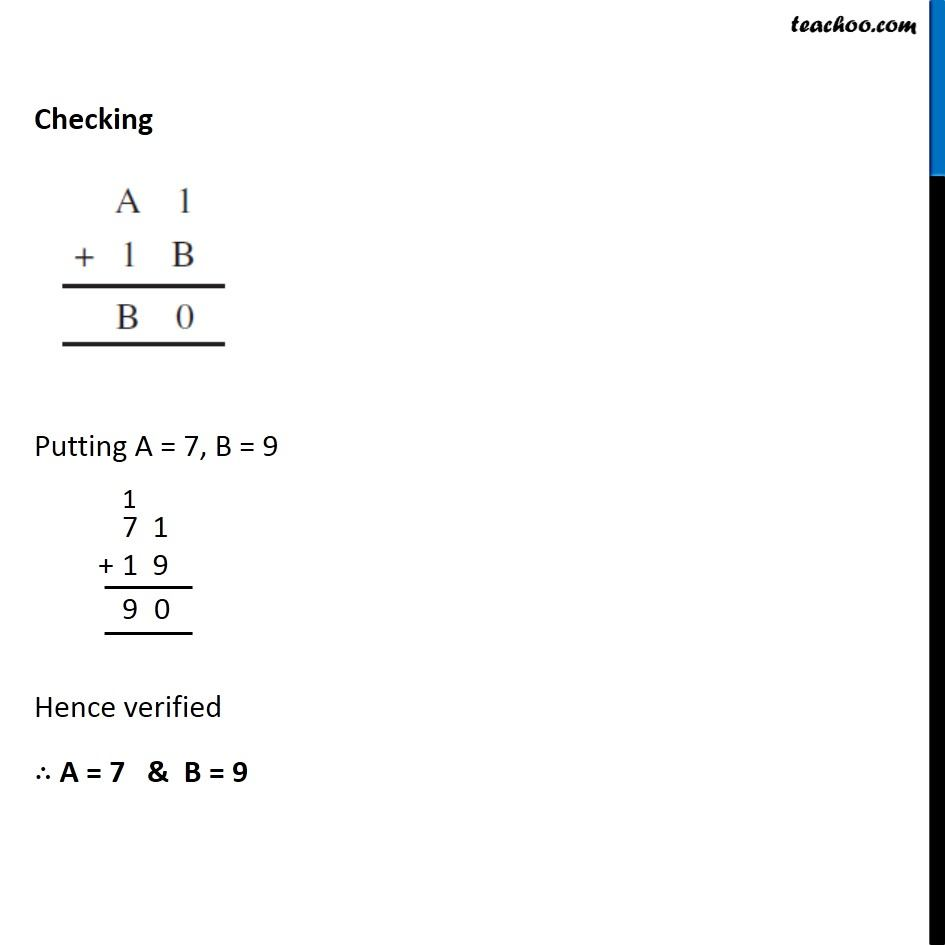1. Chapter 16 Class 8 Playing with Numbers
2. Serial order wise
3. Ex 16.1

Transcript

Ex 16.1, 8 Find the values of the letters in each of the following and give reasons for the steps involved. Here, 1 + B ends with 0 We put different values of B, and check which ends with 0 ∴ B = 9 Putting B = 9 Now, It can be written as, And, ∴ A = 7 Checking Putting A = 7, B = 9 Hence verified ∴ A = 7 & B = 9

Ex 16.1

Chapter 16 Class 8 Playing with Numbers
Serial order wise